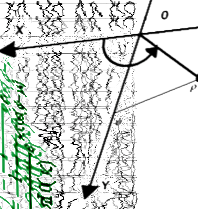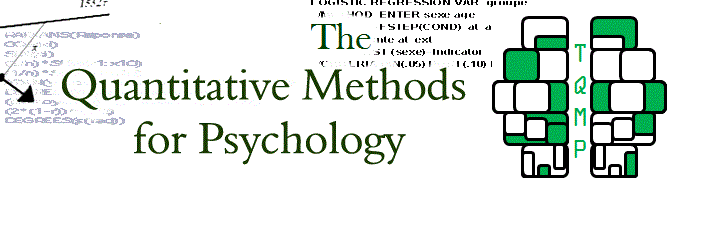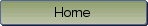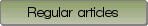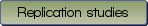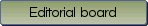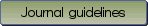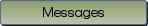The posterior probability of a null hypothesis given a statistically significant result

Bibliographic information: BibTEX format RIS format XML format APA style
Cited references information: BibTEX format APA style

Schad, Daniel J. , Vasishth, Shravan
130-99
Keywords: Null hypothesis significance testing , Bayesian inference , statistical power
Tools: R
(no sample data)   (Appendix)

When researchers carry out a null hypothesis significance test, it is tempting to assume that a statistically significant result lowers Prob(H0), the probability of the null hypothesis being true. Technically, such a statement is meaningless for various reasons: e.g., the null hypothesis does not have a probability associated with it. However, it is possible to relax certain assumptions to compute the posterior probability Prob(H0) under repeated sampling. We show in a step-by-step guide that the intuitively appealing belief, that Prob(H0) is low when significant results have been obtained under repeated sampling, is in general incorrect and depends greatly on: (a) the prior probability of the null being true; (b) type-I error rate, (c) type-II error rate, and (d) replication of a result. Through step-by-step simulations using open-source code in the R System of Statistical Computing, we show that uncertainty about the null hypothesis being true often remains high despite a significant result. To help the reader develop intuitions about this common misconception, we provide a Shiny app (https://danielschad.shinyapps.io/probnull/). We expect that this tutorial will help researchers better understand and judge results from null hypothesis significance tests.

Be informed of the upcoming issues with RSS feed:RSS